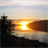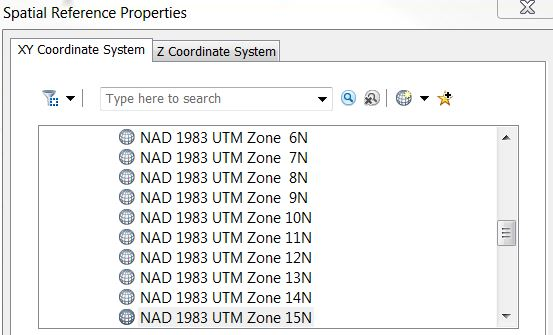# Re: Spatial Reference Code for Calculating Geometry

556
6
10-26-2018 06:33 AMby
Occasional Contributor

Randy,

Thanks for the code snippet. I’ll give it a try. If it works properly then I’ll need to incorporate into bigger script.

Regards,

Jim

6 Repliesby
Occasional Contributor

The spatial reference code 32165 does not provide the right values so I'm hoping to use a .prj file instead for the spatial reference.  In the script below is there a way to use "sr" (spatial reference) as a parameter in the arcpy.management.CalculateGeometryAttributes command line?

``````#This script will loop thru a ist of layers
#and calculate geometry from geographic to NAD_1983_UTM_Zone15_N, Feet

import arcpy
arcpy.env.overwriteOutput = 1

outrastertopoint = r"S:/General-Offices-GO-Trans/SLR-Mapping/GIS_Projects_2018/Smart_T_Line_Model/geodata/TEST_RASTERTOPOINT_TEST2.gdb/"

arcpy.env.workspace = outrastertopoint

fieldName1 = "X_coord"
fieldPrecision1 = 9
fieldAlias1 = "longitude"

fieldName2 = "Y_coord"
fieldPrecision2 = 9
fieldAlias2 = "latitude"

#sr = arcpy.SpatialReference(r"S:\General-Offices-GO-Trans\SLR-Mapping\GIS_Projects_2018\Smart_T_Line_Model\geodata\templateUTM.prj")

featureClassList = arcpy.ListFeatureClasses()

for featureClass in featureClassList:

arcpy.management.CalculateGeometryAttributes(featureClass, "X_coord POINT_X;Y_coord POINT_Y", None, None, "PROJCS['NAD 1983 UTM Zone 15N 1_1',GEOGCS['GCS_North_American_1983',DATUM['D_North_American_1983',SPHEROID['GRS_1980',6378137.0,298.257222101]],PRIMEM['Greenwich',0.0],UNIT['Degree',0.0174532925199433]],PROJECTION['Transverse_Mercator'],PARAMETER['False_Easting',500000.0],PARAMETER['False_Northing',0.0],PARAMETER['Central_Meridian',-93.0],PARAMETER['Scale_Factor',0.9996],PARAMETER['Latitude_Of_Origin',0.0],UNIT['Feet',0.3048]]")
‍‍‍‍‍‍‍‍‍‍‍‍‍‍‍‍‍‍‍‍‍‍‍‍‍‍‍‍‍``````byMVP Regular Contributor

Did this work - using a projection file?

``````sr = arcpy.SpatialReference(r"S:\General-Offices-GO-Trans\SLR-Mapping\GIS_Projects_2018\Smart_T_Line_Model\geodata\templateUTM.prj")

featureClassList = arcpy.ListFeatureClasses()

for featureClass in featureClassList:

arcpy.management.CalculateGeometryAttributes(featureClass, "X_coord POINT_X;Y_coord POINT_Y", None, None, sr)‍‍‍‍‍‍‍‍‍‍‍‍‍‍‍‍‍‍``````by
Occasional Contributor

Yes, this works in the script running in ArcGIS Pro.  Unfortunately, the x values are still coming up negative. Also, when calculating in an interactive session they come up negative as well.

When I manually calculate geometry inside of ArcMap the x values are correct (in the plus range).  Go figure.byEsri Notable Contributor

The false easting/false northing values have to use the same linear unit as the projected coordinate system itself. The well-known text string is using international feet (1 ft = 0.3048 m) but the false easting value is defined in meters.by
Occasional Contributor

Hi Melita,

I have side question.  Is there a way to project a DEM raster, from geographic to UTM15N US feet, so that the grid code z-value changes from meters to feet?  Do you have to go into the spatial reference properties and the "Z coordinate system" tab and pick from there (see image below)?  If so, what would the best parameter to use.

It seems that without setting a z value no change conversion to feet is evident after running Project Raster.Thank-You,

Jimby
Occasional Contributor

Here is the final script that seemed to do the trick:

``````This script will loop thru and calculate geometry fields
X_coord and Y_coord from geographic to NAD_83_UTM_Zone_15N_Feet

import arcpy
arcpy.env.overwriteOutput = 1

outrastertopoint = r"S:/General-Offices-GO-Trans/SLR-Mapping/GIS_Projects_2018/Smart_T_Line_Model/geodata/NSP_RASTERTOPOINT_FT.gdb/"

arcpy.env.workspace = outrastertopoint

fieldName1 = "X_coord"
fieldPrecision1 = 9
fieldAlias1 = "longitude"

fieldName2 = "Y_coord"
fieldPrecision2 = 9
fieldAlias2 = "latitude"

#Spatial reference set using .prj file.  False easting field is key to get positive values in X_coord.

sr = arcpy.SpatialReference(r"S:\General-Offices-GO-Trans\SLR-Mapping\GIS_Projects_2018\Smart_T_Line_Model\geodata\coord_sys_UTM15FT.prj")

#Loop thru list of 1,455 features

featureClassList = arcpy.ListFeatureClasses()

for featureClass in featureClassList:

arcpy.management.CalculateGeometryAttributes(featureClass, "X_coord POINT_X;Y_coord POINT_Y", None, None, sr)‍‍‍‍‍‍‍‍‍‍‍‍‍‍‍‍‍‍‍‍‍‍‍‍‍‍‍‍‍‍‍‍‍‍``````